Exercises

# Subtraction 2

Fluency with subtraction is one of the foundations of the first grade math curriculum. This guided lesson on subtraction was designed by our team of curriculum experts to reinforce first graders' understanding of subtraction, as well as boost their confidence with this operation. When finished with the lesson, kids can find extra opportunities to practice subtraction with the accompanying subtraction worksheets.
This lesson includes 5 printable learning activities.

## What's Missing? Subtraction Song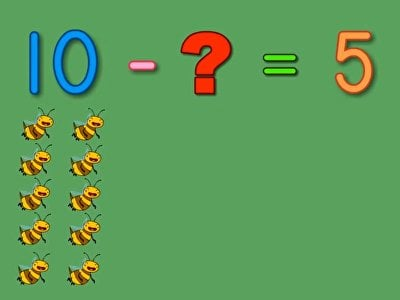Simple subtraction problems become more complex with unknowns in different spots in the equation. Help kids understand the concept of dealing with various unknowns in subtraction equations with this catchy subtraction song. From bees in the hive to caterpillars in cocoons, this song's bug theme appeals to all kinds of early learners.

## Subtraction Pizza Party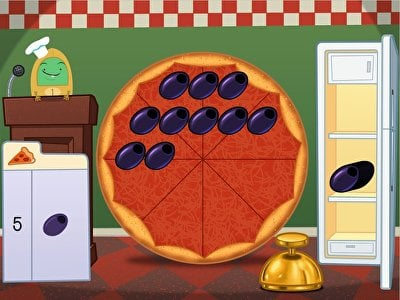Tie on your apron—it's time to fix some pizzas! In this interactive math game, kids must drag pizza toppings off of the pizza to complete subtraction problems and give each customer exactly what they order. This first grade math game uses digital manipulatives to help kids visualize the answer to problems in a concrete way, instead of relying on mental subtraction strategies.

## Subtraction Hopper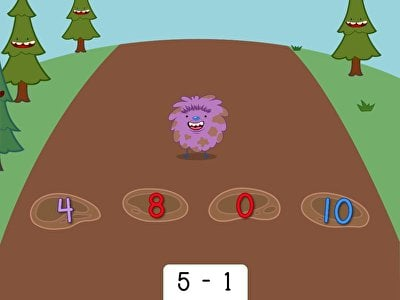Help Cuz-Cuz make a splash in this interactive subtraction game. Kids practice their mental math skills by taking away numbers in this abstract game. Perfect for honing fluent subtraction skills, this game has the educational punch and silly artwork that will please teachers, parents, and kids alike.

## Ski Race: Subtraction Within 10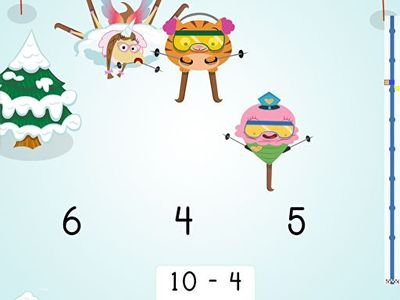Is your amateur skier ready to shred that mountain? It's not her athletic abilities, but her subtraction skills that will help guide her character down the slopes. For each single-digit subtraction problem correctly solved, her character will grab some air and get one step closer to the bottom. After a few times down the course she'll have memorized basic subtraction facts, and maybe even be first to cross the finish line!

## Match: Subtraction Facts within 10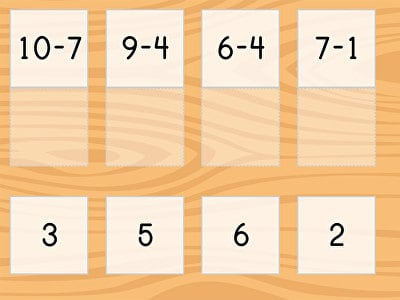Let’s practice our subtraction facts! In this math game, students must find the number that matches each subtraction statement. Perfect for first graders, Match: Subtraction Facts Within 10 is a digital exercise featuring four rounds of subtraction practice. All they have to do is find the difference that goes with each expression and identify subtraction facts within 10.

## Secret Agent Subtraction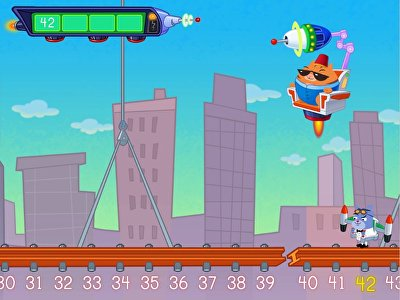Agent 00K9 needs your help to defeat El Gato! In this exciting number line game, kids must answer subtraction problems to unlock the secret code needed to defeat El Gato, and help Secret Agent Floyd avoid the shrink ray. Listen carefully to each clue before you answer; too many wrong answers, and Agent 00K9 will be shrunk!

## Asteroid Subtraction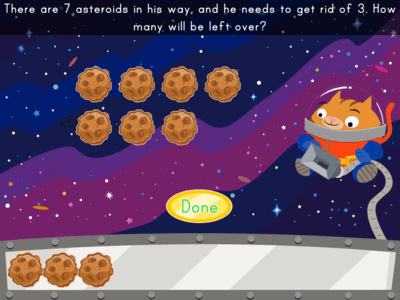Join astronaut Roly on a mission and practice subtraction, too! Help Roly clear asteroids and count how many he has left to go.

## Swamp Subtraction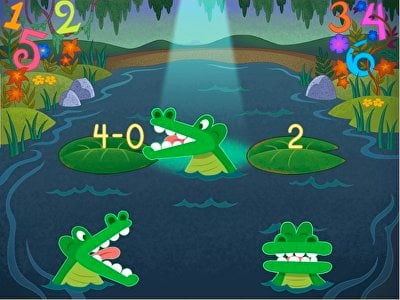These alligators are hungry for numbers! In this comparing numbers math game, kids must do mental subtraction to feed the gators the right number, using less than and greater than signs. When the number matches the difference of the subtraction problem, kids must use the equals sign to move on to the next question.

## Magical Subtraction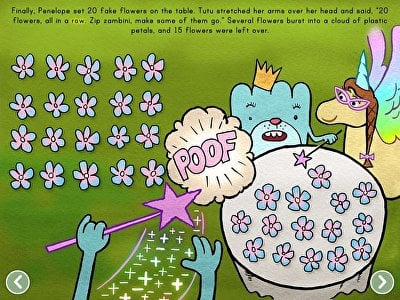Tutu's magical wand can make things disappear—but can she learn the subtraction skills she needs to bring them back? In this adorable story, kids can follow along as Tutu learns mental subtraction strategies to help her with her magic tricks, with help from her friend Penelope. Perfect for reluctant learners, this math story makes subtraction approachable and fun for every kid.

## Match: Subtraction Within 10 and Missing Factors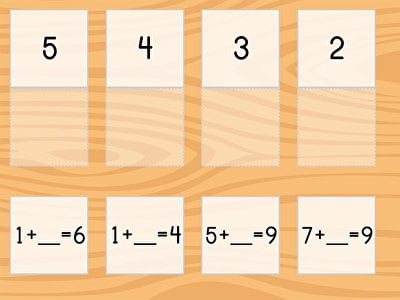What’s missing here? In this online math game, first graders look at several sets of subtraction facts that are missing one of their factors. Can they fill in the blanks with the correct numbers? Match: Subtraction Within 10 and Missing Factors gives children great subtraction practice as they puzzle out the matching sets of cards.

## Ski Racer: Practice Subtraction Within 20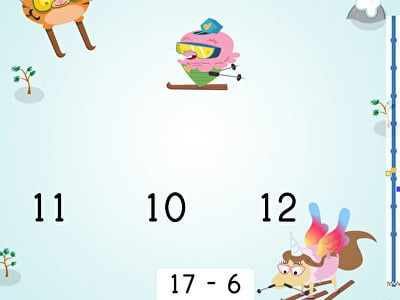Get ready to shred that slope! Players choose a Brainzy avatar to ski down the slope in this fun-filled, interactive subtraction race to the finish line. Children will use their math knowledge as they guide their ski racer down the slope by correctly solving subtraction problems. Ideal for first graders, this fast-paced learning adventure is a great way to get kids excited about practicing subtraction facts within 20.

## Match: Subtraction Within 20 and Missing Factors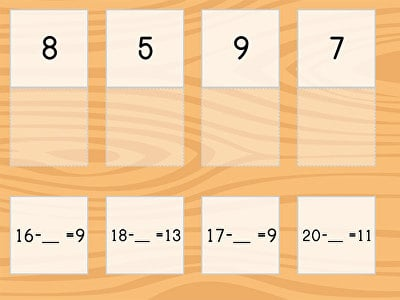Which number do you need to take away from 16 to get 8? Featuring several rounds of partially filled in subtraction facts, this math activity asks first graders to think on their feet and find the missing numbers. Match: Subtraction Within 20 and Missing Factors is a digital matching game that helps children master common subtraction facts.

## Ski Racer: Subtraction and Finding 10 Less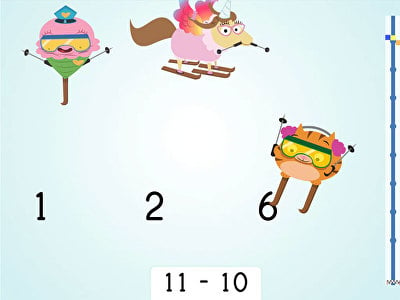Kids boost their subtraction skills while shredding the slope in Ski Racer: Subtraction and Finding 10 Less! In this fast-paced math adventure, players aim to quickly and correctly subtract 10 from two-digit numbers in order to beat the other racers to the finish line. This game is a great way for first graders to hone their mental math skills while they practice subtraction within 20.

## Ski Racer: Subtract by Multiples of 10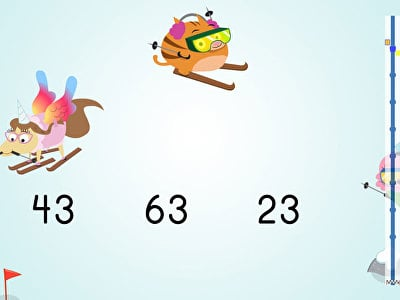Inspire your first grader to practice two-digit subtraction with this fast-moving game Ski Racer: Subtract by Multiples of 10! After choosing their favorite Brainzy character, players will help their avatar ski down the mountain and across the finish line by correctly subtracting multiples of 10 from two-digit numbers. Each time they play, children will hone their math skills and gain familiarity with foundational concepts.

## Ski Racer: Subtract by Multiples of 10 from Multiples of 10Help first graders get comfortable with multiples of 10 in this online educational game. Ski Racer: Subtract by Multiples of 10 from Multiples of 10 is a math activity that is great for students that are subtracting two-digit numbers. As they practice subtracting multiples of 10, they will build up core math skills, such as number fluency.